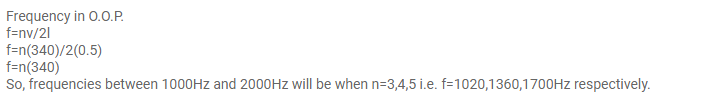# A cylindrical metal tube has a length ofQuestion:

A cylindrical metal tube has a length of $50 \mathrm{~cm}$ and is open at both ends. Find the frequencies between $1000 \mathrm{~Hz}$ and $2000 \mathrm{~Hz}$ at which the air column in the tube can resonate. Speed of sound in air is $340 \mathrm{~m} \mathrm{~s}^{-1}$.

Solution: